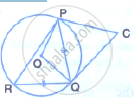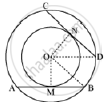Share

In the Following Figure, Pq = Qr, ∠Rqp = 68° , Pc and Cq Are Tangents to the Circle with Centre O (I) ∠Qop (Ii) ∠Qcp - ICSE Class 10 - Mathematics

ConceptNumber of Tangents from a Point on a Circle

Question

In the following figure, PQ = QR, ∠RQP  = 68° , PC and CQ are tangents to the circle with centre O(i) ∠QOP
(ii) ∠QCP

Solution

Consider two concentric circles with centres at O. Let AB and CD be two chords of the outer circle which touch the inner circle at the points M and N respectively.To prove the given question, it is sufficient to prove AB = CD.
For this join OM, ON, OB and OD.
Let the radius of outer and inner circles be R and r respectively.
AB touches the inner circle at M.
AB is a tangent to the inner circle
∴ OM ⊥ AB
⇒ BM =1/2 AB
⇒ AB = 2BM
Similarly ON ⊥ CD, and CD = 2DN
Using Pythagoras theorem in ΔOMB and ΔOND
OB^2 = OM^2  + BM^2 , OD^2 = ON^2 + DM^2
⇒ BM= sqrt(R^2 - r^2) ,DN =  sqrt(R^2 - r^2
Now,
AB = 2BM =  2 sqrt(R^2 -r^2) ,CD = 2DN =2 sqrt(R^2 -r^2)
∴ AB = CD
Hence proved.

Is there an error in this question or solution?

Video TutorialsVIEW ALL 

Solution In the Following Figure, Pq = Qr, ∠Rqp = 68° , Pc and Cq Are Tangents to the Circle with Centre O (I) ∠Qop (Ii) ∠Qcp Concept: Number of Tangents from a Point on a Circle.
S